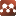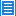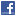Number TheoryCollatz Conjecture a New Mathematical Approach.

Authors: Darrin Taylor

A new form of mathematics is explored where a sequence of values are acted on by a set of rules (in this case the 3n+1 rules) and each digit within the values is acted on by a subordinate set of rules which produce the same values. But the digit rules allow patterns to be identified and calculations to be performed on mostly unknown values. Proves that loop length must be 13x + 18y Proves that loop is made up of segments of 8 and 11 values and names the leading digits of each value in the segments. Shows that base 4 descent is favored on average by a factor of 5. Shows that if the base 4 upper digits were always even sequence would always eventually descend. Possible future work may link the leading 0s which are infinitely even can be reflected to the least significant digits over time so that over infinity the effect approaches the always even which drives descent. Predicts loop values based on most significant base 3 digit and show quantized loop leading digits and possible pattern of increasing smallest segments. Predict general sequence based on least significant digit base 3. Predict general sequence based on least significant digit base 4.

Comments: 68 Pages. Last step of proof broke so this is merely a new mathematical framework to attack Collatz type problems

Submission history

[v1] 2019-07-09 01:25:02
[v2] 2019-08-01 03:39:38